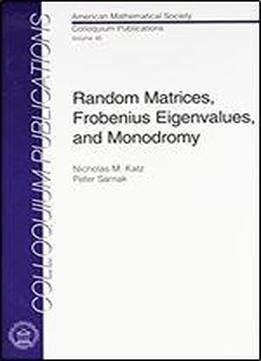# Random Matrices, Frobenius Eigenvalues, And Monodromy by Nicholas M. Katz / 1998 / English / PDF

The main topic of this book is the deep relation between the spacings between zeros of zeta and \$L\$-functions and spacings between eigenvalues of random elements of large compact classical groups. This relation, the Montgomery-Odlyzko law, is shown to hold for wide classes of zeta and \$L\$-functions over finite fields. The book draws on and gives accessible accounts of many disparate areas of mathematics, from algebraic geometry, moduli spaces, monodromy, equidistribution, and the Weil conjectures, to probability theory on the compact classical groups in the limit as their dimension goes to infinity and related techniques from orthogonal polynomials and Fredholm determinants.

views: 348